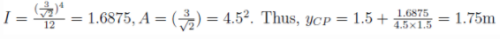Courses

# Test: Hydrostatic Force on Plane Area - 1

## 15 Questions MCQ Test Fluid Mechanics | Test: Hydrostatic Force on Plane Area - 1

Description
This mock test of Test: Hydrostatic Force on Plane Area - 1 for Civil Engineering (CE) helps you for every Civil Engineering (CE) entrance exam. This contains 15 Multiple Choice Questions for Civil Engineering (CE) Test: Hydrostatic Force on Plane Area - 1 (mcq) to study with solutions a complete question bank. The solved questions answers in this Test: Hydrostatic Force on Plane Area - 1 quiz give you a good mix of easy questions and tough questions. Civil Engineering (CE) students definitely take this Test: Hydrostatic Force on Plane Area - 1 exercise for a better result in the exam. You can find other Test: Hydrostatic Force on Plane Area - 1 extra questions, long questions & short questions for Civil Engineering (CE) on EduRev as well by searching above.
QUESTION: 1

### A cuboidal beaker is half filled with water. By what percent will the hydrostatic force on one of the vertical sides of the beaker increase if it is completely filled?

Solution:

Explanation: Hydrostatic force per unit width on a vertical side of a beaker = 1 ⁄ 2 * ρgh2, where ρ = density of the liquid and h= height of liquid column. The hydrostatic force when the beaker is completely filled = 1 ⁄ 2 ρg(2h)2 = 2ρgh2.
Thus, percentage increase in hydrostatic force =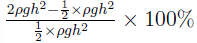= 300%.

QUESTION: 2

### By what factor will the hydrostatic force on one of the vertical sides of a beaker decrease if the height of the liquid column is halved?

Solution:

Hydrostatic force per unit width on a vertical side of a beaker = 1 ⁄ 2 * ρgh2, where ρ = density of the liquid and h= height of liquid column. Thus, if the liquid column is halved, the hydrostatic force on the vertical face will become one-fourth.

QUESTION: 3

### Equal volume of two liquids of densities ρ1 and ρ2 are poured into two identical cuboidal beakers. The hydrostatic forces on the respective vertical face of the beakers are F1 and F2respectively. If ρ1 > ρ2, which one will be the correct relation between F1 and F2?

Solution:

Explanation: Hydrostatic force per unit width on a vertical side of a beaker = 1 ⁄ 2 * ρgh2, where ρ = density of the liquid and h= height of liquid column. Thus if ρ1 > ρ2, F1 > F2 and F1 ≠ F2, when the h is constant.

QUESTION: 4

Which of the following is the correct relation between centroid (G) and the centre of pressure (P) of a plane submerged in a liquid?

Solution:

Explanation: The depth of the centroid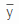and the centre of pressure yCP are related by: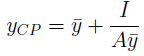where I = the moment of inertia and A= area. None of the quantities I, A andcan be negative. Thus, YCP >. For horizontal planes, I = 0, hence YCP =QUESTION: 5

A beaker contains water up to a height of h. What will be the location of the centre of pressure?

Solution:

Explanation: The depth of the centroidand the centre of pressure yCP are related by:where I = the moment of inertia and A = area. If= h ⁄ 2; I = bh3/12 ;A = bh, then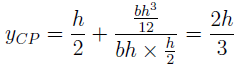QUESTION: 6

A cubic tank is completely filled with water. What will be the ratio of the hydrostatic force exerted on the base and on any one of the vertical sides?

Solution:

Explanation: Hydrostatic force per unit width on a vertical side of a beaker Fv = 1 ⁄ 2 * ρgh2, where ρ = density of the liquid and h= height of the liquid column. Hydrostatic force per unit width on the base of the beaker = Fb = ρgh * h = ρgh2. Thus, Fb : Fv = 2 : 1.

QUESTION: 7

A rectangular lamina of width b and depth d is submerged vertically in water, such that the upper edge of the lamina is at a depth h from the free surface. What will be the expression for the depth of the centroid (G)?

Solution:

Explanation: The centroid of the lamina will be located at it’s centre. ( d ⁄ 2). Thus, the depth of the centre of pressure will be h + d ⁄ 2.

QUESTION: 8

A rectangular lamina of width b and depth d is submerged vertically in water, such that the
upper edge of the lamina is at a depth h from the free surface. What will be the expression for the depth of the centre of pressure?

Solution:

Explanation: The depth of the centroidand the centre of pressure yCP are related by:where I = the moment of inertia and A = area. If= h + d ⁄ 2; I = bh3/12 ;A = bd. thus,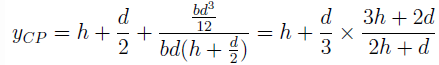QUESTION: 9

A square lamina (each side equal to 2m) is submerged vertically in water such that the upper edge of the lamina is at a depth of 0.5 m from the free surface. What will be the total water pressure (in kN) on the lamina?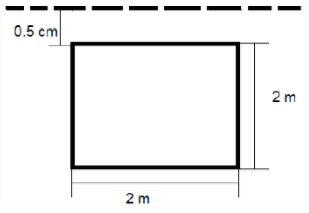Solution:

Explanation: Total liquid pressure on the lamina = F = γA, where γ = specific weight of the liquid,= depth of centroid of the lamina from the free surface, A= area of the centroid. Now, γ = 9:81 * 103 N / m3= 0.5 + 1 ⁄ 2 * 2m = 1.5 m, A = 2 * 2 m2 = 4 m2. Hence, F = 58.86 kN.

QUESTION: 10

A square lamina (each side equal to 2m) with a central hole of diameter 1m is submerged vertically in water such that the upper edge of the lamina is at a depth of 0.5 m from the free surface. What will be the total water pressure (in kN) on the lamina?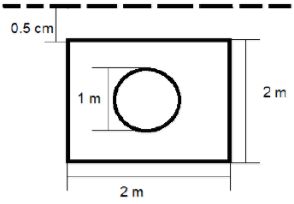Solution:

Explanation: Total liquid pressure on the lamina = F = γA, where γ = specific weight of the liquid,= depth of centroid of the lamina from the free surface, A= area of the centroid. Now, γ = 9:81 * 103 N / m3= 0.5 + 1 ⁄ 2 * 2m = 1.5 m, A = 2 * 2 – π ⁄ 4 * 12 m2 = 3.215 m2Hence, F = 47.31 kN.

QUESTION: 11

A square lamina (each side equal to 2m) is submerged vertically in water such that the upper edge of the lamina is at a depth of 0.5 m from the free surface. What will be the depth (in m) of the centre of pressure?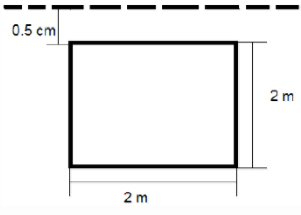Solution:

Explanation: The depth of the centroid y and the centre of pressure yCP are related by: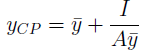where I = the moment of inertia and A = area. Now,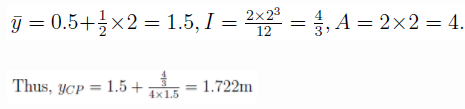QUESTION: 12

What will be the total pressure (in kN) on a vertical square lamina submerged in a tank of oil (S=0.9) as shown in the figure?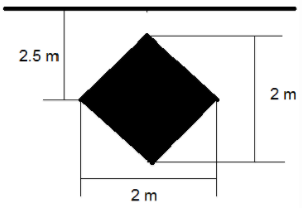Solution:

Explanation: Total liquid pressure on the lamina = F = γA, where γ = specific weight of the liquid,= depth of centroid of the lamina from the free surface, A= area of the centroid. Now, γ = 9.81 * 0.9 * 103 N / m3= 2.5m, A = 1 ⁄ 2 * 22 = 2 m2. Hence, F = 44.1 kN.

QUESTION: 13

The upper and lower edges of a square lamina of length 4 m are at a depths of 1 m and 3 m respectively in water. What will be the depth (in m) of the centre of pressure?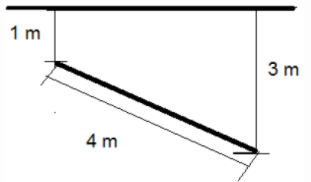Solution:

Explanation: The depth of the centroid y and the centre of pressure yCP are related by: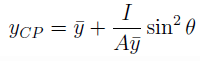where I= the moment of inertia and A = area and θ = the angle of inclination of the lamina to the horizontal. Now,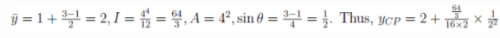= 2:17m.

QUESTION: 14

The upper and lower edges of a square lamina of length 4 m are at a depths of 1 m and 3 m respectively in water. What will be the total pressure (in kN) on the lamina?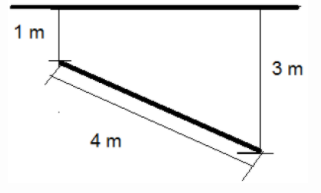Solution:

Total liquid pressure on the lamina = F = γA, where γ = specific weight of the liquid,= depth of centroid of the lamina from the free surface, A= area of the centroid. Now, γ = 9:81 * 0.9 * 103 N / m3= 1 + 3-1 / 2 = 2m, A = 4 * 4 = 16 m2. Hence, F = 313.92 kN.

QUESTION: 15

What will be the depth (in m) of the centre of pressure for a vertical square lamina submerged in a tank of oil (S=0.8) as shown in the figure?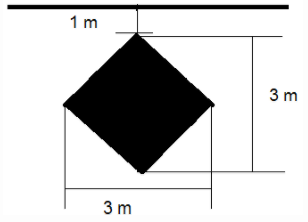Solution:

Explanation: The depth of the centroid y and the centre of pressure yCP are related by: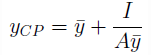where I = the moment of inertia and A = area. Each side of the lamina = 3/&sqrt;2 Now, y = 1 + 3 ⁄ 2 = 1.5,